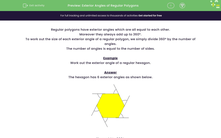# Calculate the Exterior Angles of Regular Polygons

In this worksheet, students will calculate the exterior angle of regular polygons.Key stage:  KS 3

Curriculum topic:   Geometry and Measures

Curriculum subtopic:   Derive/Use the Sum of Angles for Deduction

Popular topics:   Angles worksheets

Difficulty level:#### Worksheet Overview

Regular polygons have exterior angles that are all equal to each other.

Moreover, they always add up to 360º.

To work out the size of each exterior angle of a regular polygon, we simply divide 360º by the number of angles.

The number of angles is equal to the number of sides.

Example

Work out the exterior angle of a regular hexagon.

The hexagon has 6 exterior angles as shown below.Each exterior angle is 360º ÷ 6 = 60º

It's simple really! We can even work out the angles of any regular polygon without needing to have an image!

What size will each exterior angle of a decagon (10 sides) be?

360 ÷ 10 = 36º

Shall we have a go at the questions now?### What is EdPlace?

We're your National Curriculum aligned online education content provider helping each child succeed in English, maths and science from year 1 to GCSE. With an EdPlace account you’ll be able to track and measure progress, helping each child achieve their best. We build confidence and attainment by personalising each child’s learning at a level that suits them.

Get started